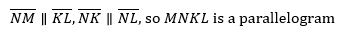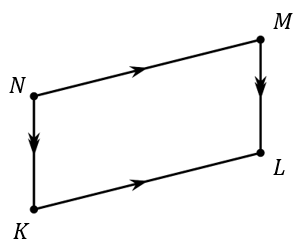# G.2.7.4 What Do We Know For Sure About Parallelograms?

The angle-side-angle theorem could be proven in a similar method to what we did for the side-angle-side theorem in the last class. Instead of spending time proving, today we are going to use the angle-side-angle theorem to prove things about parallelograms. The angle-side-angle theorem says that two triangles are congruent when two pairs of corresponding angles and the included corresponding sides are congruent. A parallelogram is a quadrilateral with two pairs of opposite parallel sides.Sketch parallelogram ABCD and then draw an auxiliary line to show how ABCD can be decomposed into 2 triangles.

Prove that the 2 triangles you created are congruent, and explain why that shows one pair of opposite sides of a parallelogram must be congruent.